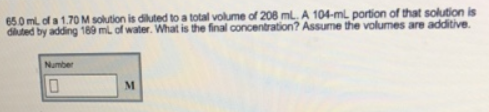# Problem: 65.0 mL of a 1.70 M solution is diluted to a total volume of 208 mL. A 104-mL portion of that solution is diluted by adding 189 ml of water. What is the final concentration? Assume the volumes are additive.

###### FREE Expert Solution
99% (58 ratings)###### Problem Details

65.0 mL of a 1.70 M solution is diluted to a total volume of 208 mL. A 104-mL portion of that solution is diluted by adding 189 ml of water. What is the final concentration? Assume the volumes are additive.